# Introduction to A* Pathfinding

This is a blog post by iOS Tutorial Team member Johann Fradj, a software developer currently full-time dedicated to iOS. He is the co-founder of Hot Apps Factory which is the creator of App Cooker. Have you ever had a game where you wanted to make monsters or players move to a particular point, while […]

#### Version

• Other, Other, Other

This is a blog post by iOS Tutorial Team member Johann Fradj, a software developer currently full-time dedicated to iOS. He is the co-founder of Hot Apps Factory which is the creator of App Cooker.

Have you ever had a game where you wanted to make monsters or players move to a particular point, while avoiding walls and obstacles?

If so, read this tutorial, and we’ll show you how you can do this with A* pathfinding!

There are already several articles on A* pathfinding on the web, but most of them are for experienced developers who already know the basics.

This tutorial takes the approach of covering the fundamentals from the ground up. We’ll discuss how the A* pathfinding algorithm works step-by-step, and include a ton of pictures and examples to diagram the process.

Regardless of what programming language or platform you are using, you should find this tutorial helpful as it explains the algorithm in a language agnostic format. Later on, we’ll have a follow-up tutorial that shows an example implementation in a Cocos2D iPhone game.

So find the shortest path to a caffeinated beverage and a tasty snack, and let’s begin! :]

## A Pathfinding Cat

Let’s imagine that we have a game where a cat wants to find a way to get a bone.

“Why in the world would a cat want a bone?!” you might think. Well in our game, this is a crafty cat and he wants to pick up bones to give to dogs, to avoid getting himself chomped! :]

So imagine the cat in the picture below wants to find the shortest path to the bone: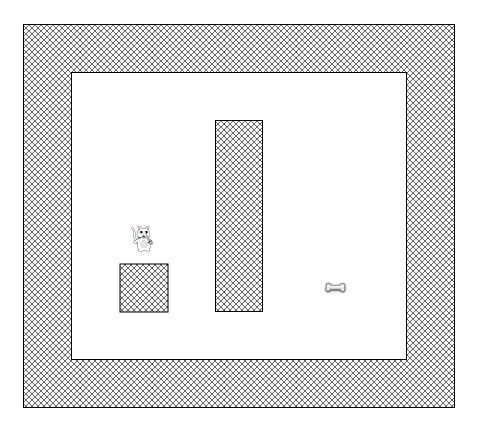Sadly, the cat can’t go straight from his current position to the bone, because there is a wall blocking the way, and he’s not a ghost cat in this game!

And the cat in this game is also quite lazy, so he always wants to find the shortest path so he’s not too tired when he gets back home to see his female ;-)

But how can we write an algorithm to figure out which path the cat should take? A* to the rescue!

## Simplifying the Search Area

The first step in pathfinding is to simplify the search area into something easily manageable.

How to do this depends on the game. For example, we could divide the search area into pixels, but that’s a granularity which is too high (and unnecessary) for our a tile-based game like this.

So instead, we will use the tile (a square shape) as unit for the pathfinding algorithm. Variants with other type of shapes are possible (such as triangles or hexagons), but the square is the best fit for our needs and is also the simplest.

Divided like that, our search area can be simply represented by a two dimensional array sized like the map it represents. So if the level is a map of 25*25 tiles, our search area will be an array of 625 squares. If we’ve divided our map into pixels, the search area would have to be an array of 640,000 squares (A tile is 32*32 pixels)!

So let’s take the screenshot we started with and divide it into tiles to represent the search area (in this simple example, 7×6 tiles = 42 tiles total):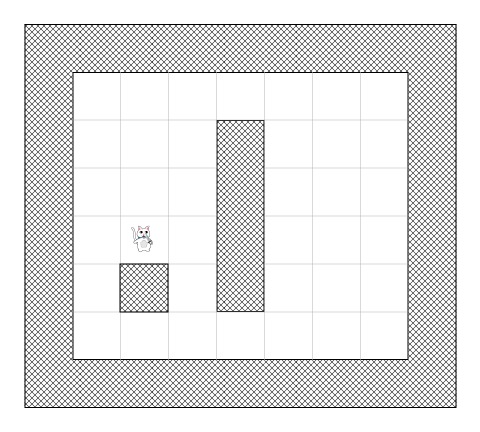## The Open and Closed Lists

Now that we’ve created a simplified search area, let’s discuss how the A* algorithm works.

In addition to being lazy, our cat does not have a good memory, so it will need two lists:

1. One to write down all the squares that are being considered to find the shortest path (called the open list)
2. One to write down the square that does not have to consider it again (called the closed list

The cat begins by adding his current position (we’ll call this starting position point “A”) to the closed list. Then, he adds all walkable tiles adjacent to his current position to the open list.

Here’s an example of what this would look like if the cat was out in the open (green representing the open list):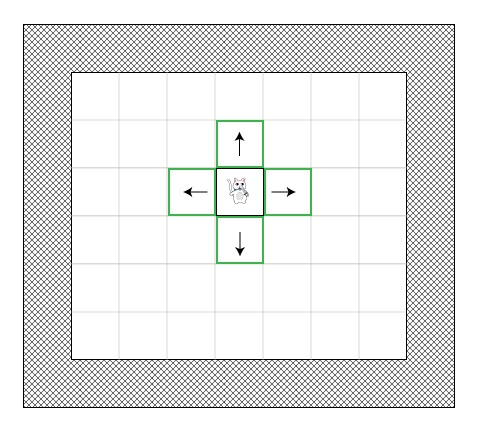Now the cat need to determine which of these options would be on the shortest path, but how can he choose?

Well in the A* path algorithm, this is done by giving a score to each square, which is called path scoring. Let’s see how it works!

## Path Scoring

We’ll give each square a score G + H where:

• G is the movement cost from the start point A to the current square. So for a square adjacent to the start point A, this would be 1, but this will increase as we get farther away from the start point.
• H is the estimated movement cost from the current square to the destination point (we’ll call this point B for Bone!) This is often called the heuristic because we don’t really know the cost yet – it’s just an estimate.

You may be wondering what we mean by “movement cost”. Well in this game it will be quite simple – just the number of squares.

However, keep in mind that you can make this different for our game. For example:

• If you allowed diagonal movement, you might make the movement cost a bit bigger for diagonal moves.
• If you had different terrain types, you might make some cost more to move through – for example a swamp, water, or a Catwoman poster ;-)

That’s the general idea – now let’s dive into more specifics about figuring out G and H.

Recall that G is the movement cost (in number of squares for this game) from the start point A to the current square.

In order to calculate G, we need to take the G of its parent (the square where we came from) and to add 1 to it. Therefore, the G of each square will represent the total cost of the generated path from point A until the square.

For example, this diagram shows two paths to two different bones, with the G score of each square listed on the square: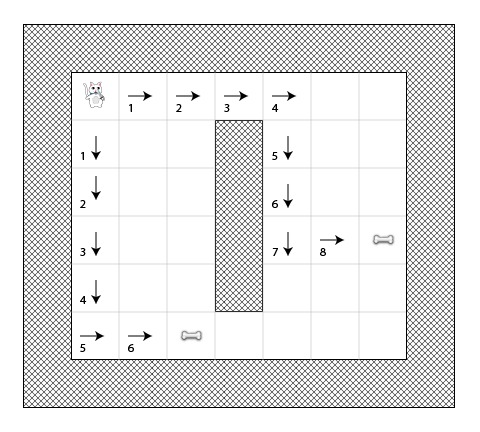Recall that H is the estimated movement cost (in number of squares for this game) from the current square to the destination point.

The closer the estimated movement cost is to the actual cost, the more accurate the final path will be. If the estimate is off, it is possible the path generated will not be the shortest (but it will probably be close). This topic is quite complex so will not be covered in this tutorial series, but I have provided a link explaining it very well at the end of the article.

To put it simply, we will use the “Manhattan distance method” (Also called “Manhattan length” or “city block distance”) that just counts the number of horizontal and vertical square remaining to reach point B without taking into account of any obstacles or differences of land.

For example, here’s a diagram that shows using the “city block distance” to estimate H (shown in black) from various starts and destinations: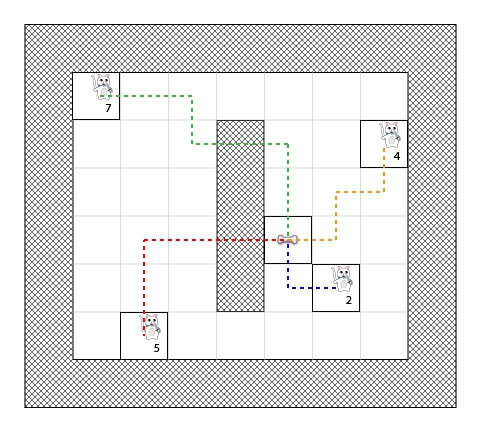## The A* Algorithm

So now that you know how to compute the score of each square (we’ll call this F, which again is equal to G + H), let’s see how the A* algorithm works.

The cat will find the shortest path by repeating the following steps:

1. Get the square on the open list which has the lowest score. Let’s call this square S.
2. Remove S from the open list and add S to the closed list.
3. For each square T in S’s walkable adjacent tiles:
1. If T is in the closed list: Ignore it.
2. If T is not in the open list: Add it and compute its score.
3. If T is already in the open list: Check if the F score is lower when we use the current generated path to get there. If it is, update its score and update its parent as well.

Don’t worry if you’re still a bit confused about how this works – we’ll walk through an example so you can see it working step by step! :]

## The Cat’s Path

Let’s go through the example of our lazy cat on the way to a bone.

In the diagrams below, I’ve listed the values for F = G + H according to the following:

• F (score for square): Top left corner
• G (cost from A to square): Bottom left corner
• H (estimated cost from square to B): Bottom right corner

Also, the arrow shows the movement direction to get to that square.

Finally, on each step the red squares indicate the closed list and the green squares indicate the open list.

OK, so let’s begin!

Step 1

In the first step, the cat determines the walkable adjacent squares to its start position (point A), computes their F scores, and adds them to its open list: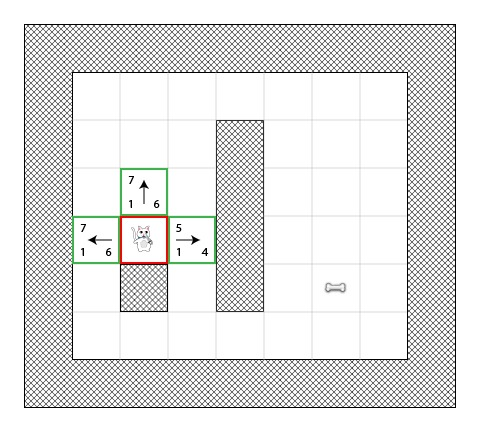You can see that the H value is listed for each square (two have 6 and one has 4). I recommend counting out the squares according to the “city block distance” to make sure you understand how that part works.

Also note that the F value (in the upper right) is just the sum of G+H (lower left and lower right).

Step 2

In the next step, the cat chooses the square with the lowest F score, adds it to the closed list, and retrieves its adjacent squares.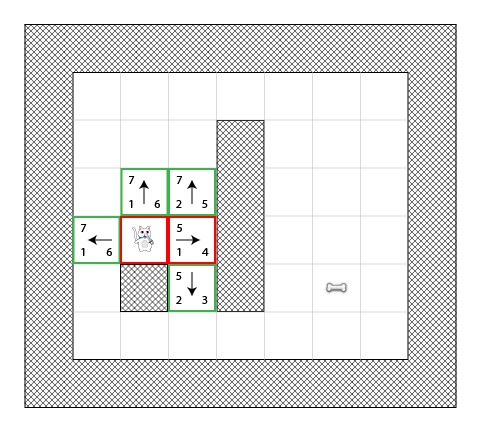So you’ll see here that the square with the lowest cost was the one that had F as 4. It tried to add any tile adjacent to this to open list (and calculate their score), except notice that it couldn’t add the cat tile (because it was already on the closed list) or the wall tile (because it wasn’t walkable).

Notice for the two new tiles added to the open list, the G values are increased by one because they are 2 tiles away from the starting point. You might also like to count out the “city block distance” to make sure you understand the H values for each of the new tiles.

Step 3

Again, we choose the tile with the lowest F score (5) and continue to iterate: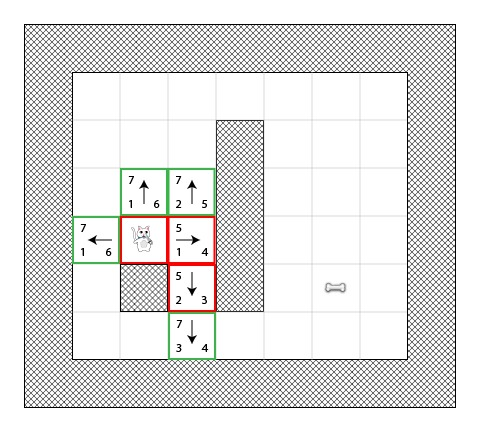In this case, there was only one possible tile to add to the open list, because one was already on the closed list and two were walls.

Step 4

Now we have an interesting case. As you can see in the previous screenshot, there are 4 squares with the same F score as 7 – what do we do?!

There are various solutions we could use, but one simple (and fast) way is to keep following the tile most recently added to the open list. So continuing on with the most recent square we have: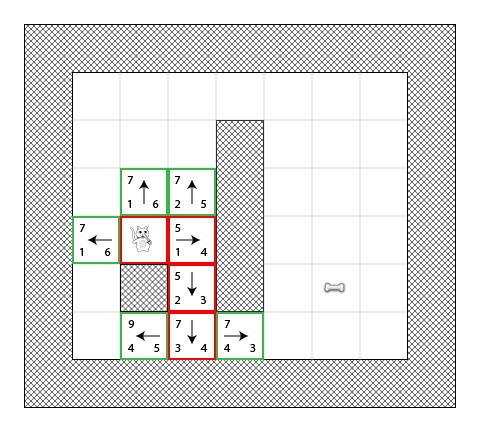This time two tiles were adjacent and walkable, and we calculate their scores as usual.

Step 5

Again we choose the tile with the lowest score (7) and in case of a tie choose the most recent: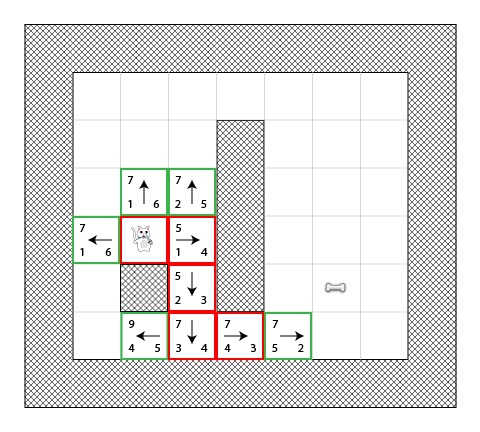Just one possibility added this time. We’re getting close!

Step 6

You get the drill by now! I bet you can guess the next step looks like the following: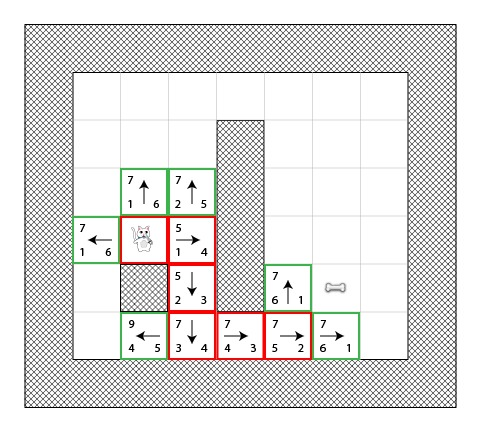We’re almost there, but this time you can see that there are actually two shortest paths to the bone we could choose between: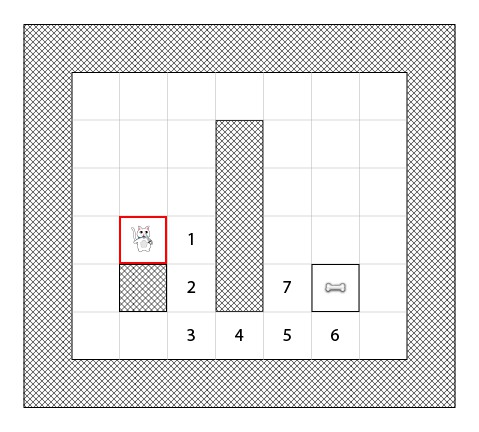In our example there is 2 differents shortest paths:

• 1-2-3-4-5-6
• 1-2-3-4-5-7

It doesn’t really matter which of these we choose, it comes down to the actual implementation in code.

Step 7

Let’s iterate one more time from one of these squares: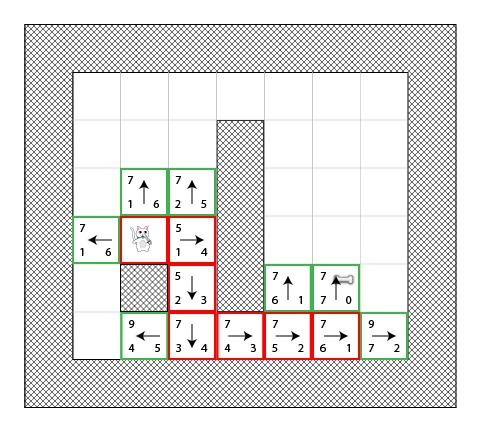Aha, the bone is in the open list!

Step 8

In the case where the target square is in the open list, the algorithm adds it to the closed list: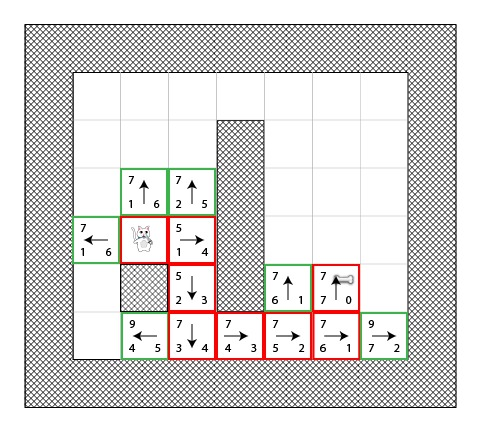Then all the algorithm has to do is go backwards to figure out the final path!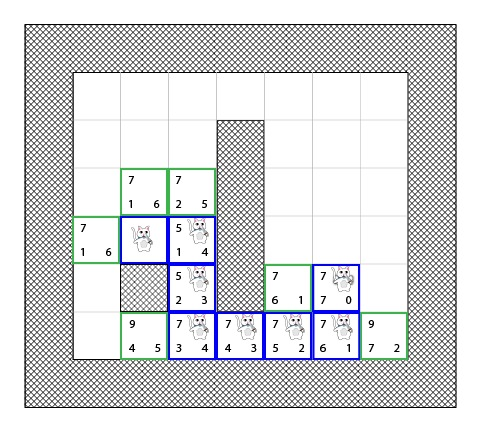## A Non-Visionary Cat

In the example above, we saw that while the cat was finding the shortest path, he often chose the better square (the one along his future shortest path) – kind of like he was a visionary cat!

But what would happen if the cat was not extralucid and always chose the first square added to his list?

Here’s an illustration that shows what would all the squares that would have been used in this process. You’ll see that the cat tries more squares, but he still finds a shortest path (not the same as previous, but another equivalent):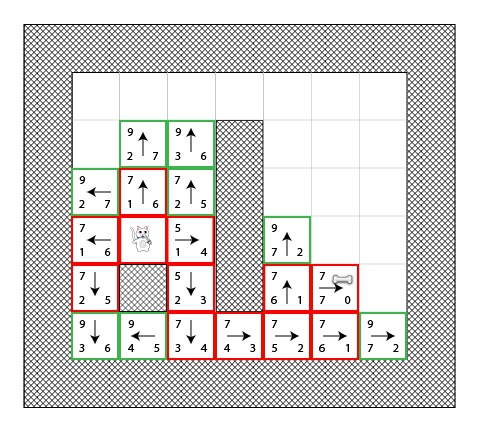The red squares in the diagram don’t represent the shortest path, they just represent the squares that have been chosen as an “S” square at some point.

I recommend looking at the above diagram and trying to go through it. This time, whenever you see a tie always choose the “worst” way to go. You’ll see that you still end up with the shortest path!

So you can see that it’s no problem to follow the “wrong” square, you’ll still end up with the shortest path even if it takes more iterations.

So in our implementation, we’ll add squares to the open list with the following algorithm:

• Adjacent squares will be returned in that order: top / left / bottom / right.
• A square will be added in the open list after all the squares that have the same score (so the first added square will be the first picked by the cat).

Here’s a diagram of the backtracking: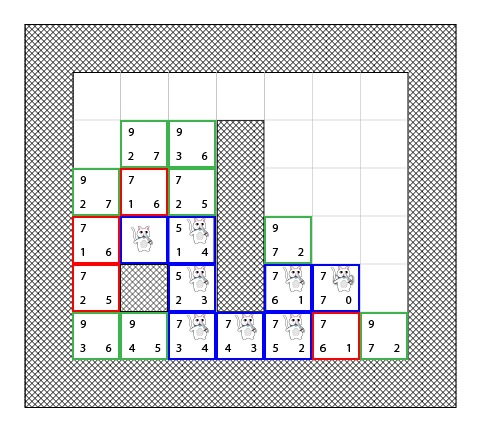The shortest path is construct by starting by the final destination and go backward from parent to parent (example: at the final destination we can see that the arrow heading right, so the parent of that square is at its left).

To conclude, we can synthesize the cat process with the following pseudo code. This is Objective-C, but you could implement this in any language:

```[openList add:originalSquare]; // start by adding the original position to the open list
do {
currentSquare = [openList squareWithLowestFScore]; // Get the square with the lowest F score

[openList remove:currentSquare]; // remove it to the open list

if ([closedList contains:destinationSquare]) { // if we added the destination to the closed list, we've found a path
// PATH FOUND
break; // break the loop
}

if ([closedList contains:aSquare]) { // if this adjacent square is already in the closed list ignore it
continue; // Go to the next adjacent square
}

if (![openList contains:aSquare]) { // if its not in the open list

// compute its score, set the parent

} else { // if its already in the open list

// test if using the current G score make the aSquare F score lower, if yes update the parent because it means its a better path

}
}

} while(![openList isEmpty]); // Continue until there is no more available square in the open list (which means there is no path)
```

Are you getting excited to implement this yet?! In the next tutorial, we’ll do exactly that!

## Where To Go From Here?

Congrats, you now know the basics of A* pathfinding! If you want ot learn more from here, I recommend reading Amit’s A* Pages.

In the next tutorial in the series, we’ll implement the A* algorithm in a simple Cocos2D tile mapped game!

In the meantime, if you have any questions about the A* algorithm in general, please join the forum discussion below!This is a blog post by iOS Tutorial Team member Johann Fradj, a software developer currently full-time dedicated to iOS. He is the co-founder of Hot Apps Factory which is the creator of App Cooker.